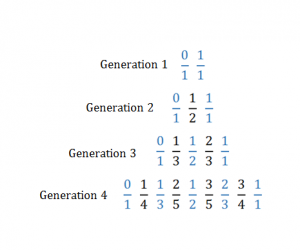### On Farey Sequences

Think back to when you were in elementary school and first learned about fractions. You learned that there is a numerator and denominator, and then you learned about multiplication. Multiplication was simple. All you need to do is multiply the numerators and then multiply the denominators to get the numerator and denominator, just as follows:$\frac{a}{b} \times \frac{c}{d} = \frac{a \times c}{b \times d}$

Naturally, when it turned to addition, we thought it would be the same. Just add the numerators together and the denominators together! Alas, when we brought this up to our teachers and parents, they told us we were wrong. The “correct” way to add two fractions is to find the common denominator and then add the two numerators. As follows:$\frac{a}{b} + \frac{c}{d} = \frac{ad}{bd} + \frac{bc}{bd} = \frac{ad+bc}{bd}$

From a young age we were taught to conform to this definition of addition, and after a while this definition seemed to make sense. But what if for a second we went with our original idea? What happens then? First let’s denote, for the purposes of this article,$\oplus$ as the addition function described above as defined by:$\frac{a}{b} \oplus \frac{c}{d} = \frac{a+c}{b+d}$

First, lets look at a few examples. We have$\frac{1}{2} \oplus \frac{2}{3} = \frac{3}{5}$ and$\frac{1}{5} \oplus \frac{2}{4} = \frac{3}{9}$. By the traditional definition of addition, these equations make no sense. Of course they don’t. This the point where our third grade teacher tells us how foolish we were and how we should accept the harder, but more correct definition of addition. But is it really the case that there is nothing noteworthy in the above statements?

After some searching, notice how when you add two fractions together with the$\oplus$ function, the result is always in between the two inputs numerically. For example, looking at the first equation,$\frac{3}{5}$ is larger than$\frac{1}{2}$ but smaller than$\frac{2}{3}$.

But this isn’t that interesting. After some thought, this result is fairly obvious. The$\oplus$ function still seems like a nonsense function. However this fact is the foundation of something very interesting called Farey sequences.

To explain this concept consider the fractions$\frac{0}{1}$ and$\frac{1}{1}$. Denote this generation 1. Now take these two and add them with the$\oplus$ function. Now we have the fractions$\frac{0}{1} \frac{1}{2} \frac{1}{1}$. Denote this generation 2.

Now, between each pair of consecutive terms in generation 2, apply the$\oplus$ operator on them, and place the resulting fraction in between the two parent fractions. Remember to reduce, of course!

You obtain the list$\frac{0}{1} \frac{1}{3} \frac{1}{2} \frac{2}{3} \frac{1}{1}$. Denote this list generation 3. You can keep on doing this to generate any generation n. Notice four generations illustrated in the chart below. Do you notice an interesting pattern?You can keep generating new generations by using the operator between two terms of the previous sequence. Blue represents the parent terms pulled from the previous generation.

Now—stay with me here—remove all fractions with denominators greater than the generation number.

It turns out that for each generation n, the list of fractions is exactly the list of all fractions between 0 and 1 with denominator at most n in increasing order—which is exactly what a Farey sequence is defined as!

It turns out that using the$\oplus$ function is one of the fastest ways for a computer (or a human) to generate a complete list of increasing rationals with denominators all less than a certain value.

From such a nonsensical and strange thing such as the$\oplus$ function, we are able to find such an interesting thing as the Farey Sequence!

We need not be limited by the traditional ideas of mathematics. Any idea, however deviant, can be explored and analyzed. The beauty of mathematics is our ability to take such strange new ideas, and to find order and patterns in the universes that these ideas create.

| September 11th, 2016 | Posted in Uncategorized |